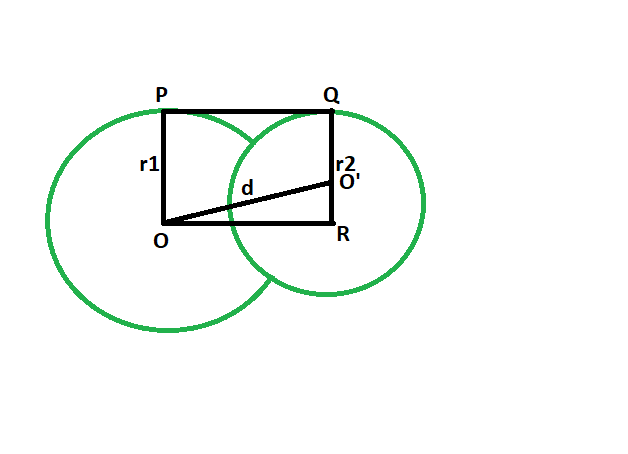Related Articles

# Length of direct common tangent between two intersecting Circles

• Last Updated : 19 Mar, 2021

Given two circles, of given radii, have there centres a given distance apart, such that the circles intersect each other at two points. The task is to find the length of the direct common tangent between the circles.
Examples:

```Input: r1 = 4, r2 = 6, d = 3
Output: 2.23607

Input: r1 = 14, r2 = 43, d = 35
Output: 19.5959```Approach:

• Let the radii of the circles be r1 & r2 respectively.

• Let the distance between the centers be d units.

• Draw a line OR parallel to PQ

• angle OPQ = 90 deg
angle O’QP = 90 deg
{ line joining the centre of the circle to the point of contact makes an angle of 90 degrees with the tangent }

• angle OPQ + angle O’QP = 180 deg
OP || QR

• Since opposite sides are parallel and interior angles are 90, therefore OPQR is a rectangle.

• So OP = QR = r1 and PQ = OR = d

• In triangle OO’R
angle ORO’ = 90
By Pythagoras theorem
OR^2 + O’R^2 = (OO’^2)
OR^2 + (r1-r2)^2 = d^2

• so, OR^2= d^2-(r1-r2)^2
OR = √{d^2-(r1-r2)^2}Below is the implementation of the above approach:

## C++

 `// C++ program to find``// the length of the direct``// common tangent between two circles``// which intersect each other``#include ``using` `namespace` `std;` `// Function to find the length of the direct common tangent``void` `lengtang(``double` `r1, ``double` `r2, ``double` `d)``{``    ``cout << ``"The length of the direct"``         ``<<``" common tangent is "``         ``<< ``sqrt``(``pow``(d, 2) - ``pow``((r1 - r2), 2))``         ``<< endl;``}` `// Driver code``int` `main()``{``    ``double` `r1 = 4, r2 = 6, d = 3;``    ``lengtang(r1, r2, d);``    ``return` `0;``}`

## Java

 `// Java program to find``// the length of the direct``// common tangent between two circles``// which intersect each other``class` `GFG``{` `    ``// Function to find the length of``    ``// the direct common tangent``    ``static` `void` `lengtang(``double` `r1, ``double` `r2, ``double` `d)``    ``{``        ``System.out.println(``"The length of the direct"``                ``+ ``" common tangent is "``                ``+ (Math.sqrt(Math.pow(d, ``2``) -``                    ``Math.pow((r1 - r2), ``2``))));``    ``}` `    ``// Driver code``    ``public` `static` `void` `main(String[] args)``    ``{``        ``double` `r1 = ``4``, r2 = ``6``, d = ``3``;``        ``lengtang(r1, r2, d);``    ``}``}` `/* This code contributed by PrinciRaj1992 */`

## Python3

 `# Python program to find``# the length of the direct``# common tangent between two circles``# which intersect each other`` ` `# Function to find the length of``# the direct common tangent``def` `lengtang(r1, r2, d):``    ``print``(``"The length of the direct common tangent is "``        ``,((d``*``*` `2``) ``-` `((r1 ``-` `r2)``*``*` `2``))``*``*``(``1``/``2``));` ` ` `# Driver code``r1 ``=` `4``; r2 ``=` `6``; d ``=` `3``;``lengtang(r1, r2, d);` `# This code has been contributed by 29AjayKumar`

## C#

 `// C# program to find``// the length of the direct``// common tangent between two circles``// which intersect each other``using` `System;` `class` `GFG``{` `    ``// Function to find the length of``    ``// the direct common tangent``    ``static` `void` `lengtang(``double` `r1, ``double` `r2, ``double` `d)``    ``{``        ``Console.WriteLine(``"The length of the direct"``                ``+ ``" common tangent is "``                ``+ (Math.Sqrt(Math.Pow(d, 2) -``                    ``Math.Pow((r1 - r2), 2))));``    ``}` `    ``// Driver code``    ``public` `static` `void` `Main(String[] args)``    ``{``        ``double` `r1 = 4, r2 = 6, d = 3;``        ``lengtang(r1, r2, d);``    ``}``}` `/* This code contributed by PrinciRaj1992 */`

## PHP

 ``

## Javascript

 ``
Output:
`The length of the direct common tangent is 2.23607`

Attention reader! Don’t stop learning now. Get hold of all the important DSA concepts with the DSA Self Paced Course at a student-friendly price and become industry ready.  To complete your preparation from learning a language to DS Algo and many more,  please refer Complete Interview Preparation Course.

In case you wish to attend live classes with experts, please refer DSA Live Classes for Working Professionals and Competitive Programming Live for Students.

My Personal Notes arrow_drop_up